cpp的内容整理。

# 1. 基本概念

## 1.1 string和char数组的区别

string的字面值是char数组，可以将string理解为`vector<char>`

``````string s5 = "hello" + ", ";  // 错误，两个运算对象都不是 string
string s1=s2+"1"; //ok
``````

## 1.2 unsigned类型

`unsigned`类型是`unsigned int`的简写。范围0-255，超出结果会wrap around环绕。

``````int x=1e10; //得到一个随机值
unsigned s=257 //得到1
``````

``````for (unsigned u = 10; u >= 0; --u)
std::cout << u << std::endl //死循环
``````

## 1.3 变量初始化方法

``````int a=0;
int a={0};
int a(0);
int a{0};
``````

``````long double pi=3.1415926535;
int a{pi}; //error
int a=pi; //ok,but loss information
``````

## 1.4 引用和指针

``````double dval =3.14;
void* pv = &dval;//pv还是 void*类型
``````

• 都是复合类型（基本类型+改造符或声明符）
• 都是间接访问

• 指针本身就是一个对象而引用不是，所以指针可以被赋值和拷贝，而引用不能。指针不需要定义时初始化
• 一个指针可以指向好几个不同的对象，而引用则被绑死

## 1.5 类和结构体的区别

class

• class 在堆中分配空间，栈中保存的只是引用
• 默认是private

struct

• struct 在栈中分配空间
• 默认是public

## 1.8 IO刷新缓冲区的原因

• 程序跑完，从main中返回
• 缓冲区满
• 特殊符号endl，flush，ends结尾
• 使用unitbuf操作符，默认情况下，unitbuf为cerr默认设置

## 1.10 生成可执行文件的过程

• 用编辑器编写源代码，如.c文件。
• 用编译器编译代码生成目标文件，如.o。
• 用链接器连接目标代码生成可执行文件，如.exe。

## 1.12 int (*s)(int) 表示的是什么？

`int (*s)(int)` 函数指针数组，每个指针指向一个`int func(int param)`的函数。

``````int (*fp)(int a);
//fp可以指向如下函数
int myFunction(int a){...}
``````

## 1.13 联合

``````union abc {
int i;
char m;
};
union abc a;
a.i = 10;
``````

# 2. 关键词和操作符

## 2.1 extern

CPP初始化通常分为两个部分：

• 声明，让程序知道他的名字
• 定义，实现实体。

`extern`关键词来进行声明，这样就不用进行显示初始化。

``````extern int a;
int b;
``````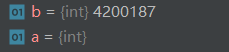## 2.2 constexpr

``````const int a=10; //constant expression
const int b=get_size(); //non a constant expression
``````

``````class Account
{
public:
static double rate() { return interestRate; }
static void rate(double);
private:
static constexpr int period = 30;// period is a constant
}
``````

## 2.3 sizeof

``````vector<int> a{1,2,3};
auto m = sizeof a; // type is unsigned_int64, m = 32
auto n = sizeof a; //type is the same as above, n=4;
``````

## 2.4 mutable

``````char Screen::get() const {
return _screen[_cursor];
}
``````

``````class Screen {
public:
void some_member() const;
private:
mutable size_t access_ctr;
}
void Screen::some_member() const {
++access_ctr;
}
``````

## 2.5 explicit

``````class A{
public:
explicit A(int size){}
explicit A(const Explicit& ins){}
};

int main(){
A test0(15);
A test1 = 10;// 无法调用
A test5 = test0;//无法调用
//如果去掉explicit,就都可以调用。
}
``````

## 2.6 inline

• 相当于把内联函数里面的内容写在调用内联函数处；
• 相当于不用执行进入函数的步骤，直接执行函数体；

## 2.8 typedef struct

``````struct Student{
int a;
}stu1;//stu1是一个变量

typedef struct Student2{
int a;
}stu2;//stu2是一个结构体类型

auto m = stu1.a; //ok
auto n = stu2.a; //error
stu2 st;
auto n = st.a;//ok
``````

# 3. new

## 3.1 new和delete的执行过程

new的步骤：

1. 分配一块足够大的原始的未命名的内存空间
2. 调用相应的构造函数构造对象，为其传入初值
3. 构造结束后返回该对象的指针

delete步骤：

1. 调用对象的析构函数
2. 编译器释放内存空间

## 3.2 动态数组

``````int* p = new int[obj.getlen()]; // new运算符返回第一个元素的地址。
delete [] p;          // 释放整个数组，new如果带[] 则delete也需要带[]
``````

• 避免使用delete来释放不是new分配的资源；
• 不要对同一资源delete两次
• 使用new[]分配资源时，应使用delete[]来释放
• 可以对空指针delete

## 3.3 智能指针

• shared_ptr，允许多个指针指向同一个对象
• unique_ptr，对对象拥有唯一所有权，可以移动但不能复制
• weak_ptr，和shared_ptr搭配使用检测他是否空悬，每次使用时不会影响指向对象的引用计数

``````shared_ptr<string> p1=make_shared<string>("hello");
shared_ptr<int> p2=make_shared<int>();//empty
shared_ptr<int> p3(new int(10));
``````

reset函数作用

``````p = std::make_shared<int>(5);
p.reset(new int(5));//等价

p = nullptr;
p.reset();//等价
``````

``````unique_ptr<int> p2(new int(42));
``````

``````unique_ptr<int> p1=new int(42);
auto p2(p1);//error
auto p3 = p1;//error
``````

``````unique_ptr<int> p1(new int(42));
unique_ptr<int> p2(p1.release());
unique_ptr<int> p3(new int(10));
p3.reset(p2.release);
``````

``````auto p2(p1.release()); //int*
``````

``````unique_ptr<int> help(A a){
unique_ptr<A> pa(new A(a));
return pa;
}
``````

## 3.4 new和malloc区别

1.返回类型

new操作符分配成功是返回的指针类型和预期结果相同，无序进行转化，而malloc返回为void*，需要强制转化

``````char* p1=static_cast<char>(malloc(10));
``````

2.失败返回

3.内存位置

new从自由内存区申请(free store)，malloc从堆上申请。自由内存区可以是堆也可以不是，这取决于实现细节，也可以是静态内存区。

4.是否指定内存大小

new分配时无须指定内存块的大小，编译器自行计算，而malloc则需要显式指出内存大小。

5. 是否可以被重载

new和delete可以，malloc不可以

6. 重新分配内存

7. 构造函数

new会调用构造函数和析构函数，而malloc只是分配内存空间。

8. 处理数组

C++提供了new[]和delete[]来专门处理数组。注意delete[]要与new[]配套使用，不然会找出数组对象部分释放的现象，造成内存泄漏。

``````A * ptr = new A;//分配10个A对象
delete [] ptr;
``````

malloc需要手动指定数组大小：

``````int * ptr = (int *) malloc( sizeof(int)* 10 );//分配一个10个int元素的数组
``````

9. 是否可以互相调用

new可以重载调用malloc，而malloc反过来则不行。

# define是宏定义，只是换了一个名字。而使用const时编译器会对const进行类型检查。

## 4.2 const用处

（1）变量被指定为const

``````const int bufsize =512;
bufsize =100; //error
``````

（2）引用被指定为const

``````const int ci=1024;
const int &rci = ci;
rci =42; //error
``````

``````int i=43;
const int &ri=i;
ri=10; //error
i =10; //ok
``````

（3）指针指定为const

``````int m=10;
const int* ptr = &m; //ok, const pointer to non-const varible
m = 20; //ok
``````

## 4.4 什么是顶层常量和底层常量？

``````int i = 0;
int * const p1 = &i;  //顶层const
int *x = p1;  // 正确，可以忽略p1的顶层const
int n = *p1;  // 正确

int i = 0;
const int * p1 = &i; //底层const
int *x = p1; // 错误，*x不具备底层const资格
const int *x2 = p1; // 正确，具备底层const资格
``````

## 4.5 类的成员函数使用const

``````const int FunctionConst::getValue(){
return value;//返回值是 const, 使用指针时很有用.
}

int FunctionConst::getValue2() const{
//此函数不能修改class FunctionConst的成员函数 value
value = 15;//错误的, 因为函数后面加 const
return value;
}
``````

# 5.类与对象

## 5.1 类和结构体区别

• class
• class 是引用类型，它在堆中分配空间，栈中保存的只是引用
• 默认是private
• struct
• struct 是值类型，它在栈中分配空间
• 默认是public

## 5.2 构造函数中const和引用的初始化

``````class A{
public:
A(int ii);
private:
int i;
const int ci;
int& ri;
}
A::A(int ii){
i = ii; //ok
ci = ii; //error;
ri = ii; //error
}
``````

const和引用的初始化必须在创建的时候就要完成，所以我们需要用列表初始化：

``````A::A(int ii):i(ii),ci(ii),ri(i){}
``````

## 5.3 静态成员

• 作用域符， `r=Account::rate();`

• 传统办法（对象，引用，类指针）

``````Account ac1;
Account ac2;
r=ac1.rate();
r=ac2->rate();
``````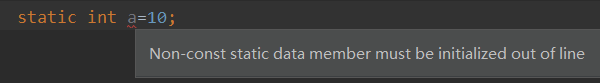``````class Account
{
public:
static double rate() { return interestRate; }
static void rate(double);
private:
static constexpr int period = 30;// period is a constant
}
``````

## 5.4 类的特殊成员函数

``````class Foo{
public:
Foo();//default
Foo(Foo const&);//copy
}
``````

``````class Foo{
public:
Foo& operator=(Foo const&);
}
``````

``````class A{
public:
int i;
A(int const& _i) :i(_i) {}
~A(){
cout << i;
}
};//先输出i，再销毁
``````

## 5.5 析构函数注意事项

``````//深拷贝
HasPtr& operator=(HasPtr const& rhs){
this->ps = new size_t[num];
this->num = rhs.num;
for(int i=0;i<num;i++)
this->ps[i]=rhs.ps[i];
return this*;
}
//浅拷贝
HasPtr& operator=(HasPtr const& rhs){
this->num=rhs.num;
this->ps = rhs.ps;
return this*;
}
``````

``````int* p1 = new int(5);
int* p2 = p1;
delete p1; //野

shared_ptr<int> p3 = make_shared<int>(5);
auto p4 = p3;
p3.reset();//ok
``````

## 5.6 合成构造函数

``````class Sales_data{
public:
Sales_data(const Sales_data&);
private:
std::string bookNo;
int units_sold = 0;
double revenue = 0.0;
};

//与Sales_data的合成拷贝构造函数等价
Sales_data::Sales_data(const Sales_data &orig) :
bookNo(orig.bookNo), //使用string的拷贝构造函数
units_sold(orig.units_sold),    //拷贝orig.units_sold
revenue(orig.revenue)    //拷贝orig.revenue
{}
}
``````

## 5.8 如何区分移动和拷贝的重载？

``````void push_back(X const&);
void push_back(X&&);
``````

``````class Foo{
public:
void sorted()&&    {cout << "&&"<<endl;}
void sorted()&    {cout << "&" << endl;}
};
Foo&& retVal(){
Foo f;
return std::move(f);
}
Foo& retFoo(){
Foo f;
return f;
}

int main(){
retVal().sorted(); //&&
retFoo().sorted(); //&
}
``````

## 5.9 this指针

``````void Student::setname(char *name){
this->name = name;
}
``````

• 类的成员函数访问
• 友元函数

# 6. 面向对象

• 封装：分离接口和实现过程
• 继承：应用类之间的相似性
• 多态：函数复用

## 6.2 虚函数

• 编译时多态，重载
• 运行时多态，重写（重写是子类对父类的允许访问的方法的实现过程进行重新编写

``````class BaseItem {
public:
virtual void itemMethod()=0;
};

class ItemA :public BaseItem { public:void itemMethod() override { cout << "ItemA"<<endl; } };
class ItemB :public BaseItem { public:void itemMethod() override { cout << "ItemB" << endl; } };
class ItemC :public BaseItem { public:void itemMethod() override { cout << "ItemC" << endl; } };

int main(){
vector<shared_ptr<BaseItem>> ItemCollection;
shared_ptr<BaseItem> p;

//基类指针指向派生类！
p.reset(new ItemA());
ItemCollection.push_back(p);
p.reset(new ItemB());
ItemCollection.push_back(p);
p.reset(new ItemC());
ItemCollection.push_back(p);

for (auto it : ItemCollection) {
it->itemMethod();
}
}
``````

C++11中允许使用override关键词显式注明虚函数的重写，如果重写失败就报错(不加的话会误以为一个新函数)(IDE推荐使用override)

``````struct B{
virtual void f1(int) const;
virtual void f2();
void f3();
};
struct D1 : B {
void f1(int) const override;    // ok: f1 matches f1 in the base
void f2(int) override;      // error: B has no f2(int) function
void f3() override;     // error: f3 not virtual
}
``````

## 6.3 虚函数表

``````class A {
public:
virtual void vfunc1();
virtual void vfunc2();
void func1();
void func2();
private:
int m_data1, m_data2;
};
``````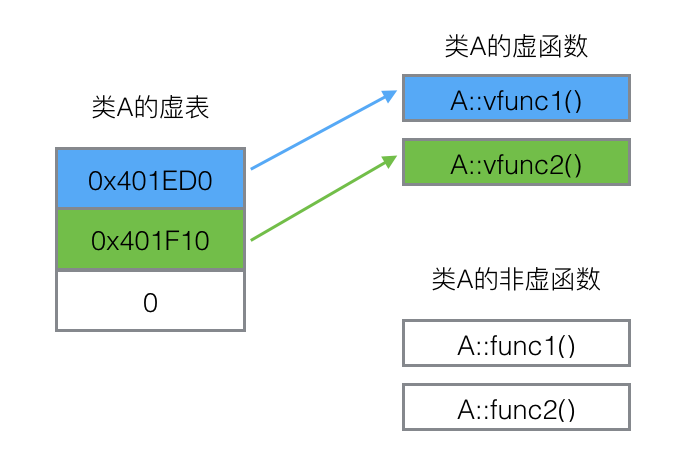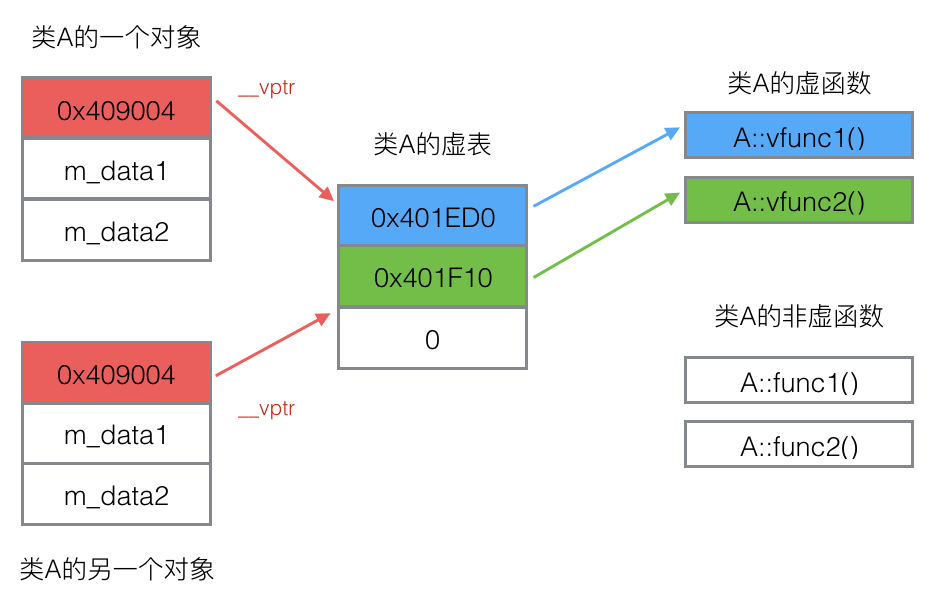``````class A {
public:
virtual void vfunc1();
virtual void vfunc2();
};

class B : public A {
public:
virtual void vfunc1();
};

class C: public B {
public:
virtual void vfunc2();
};
``````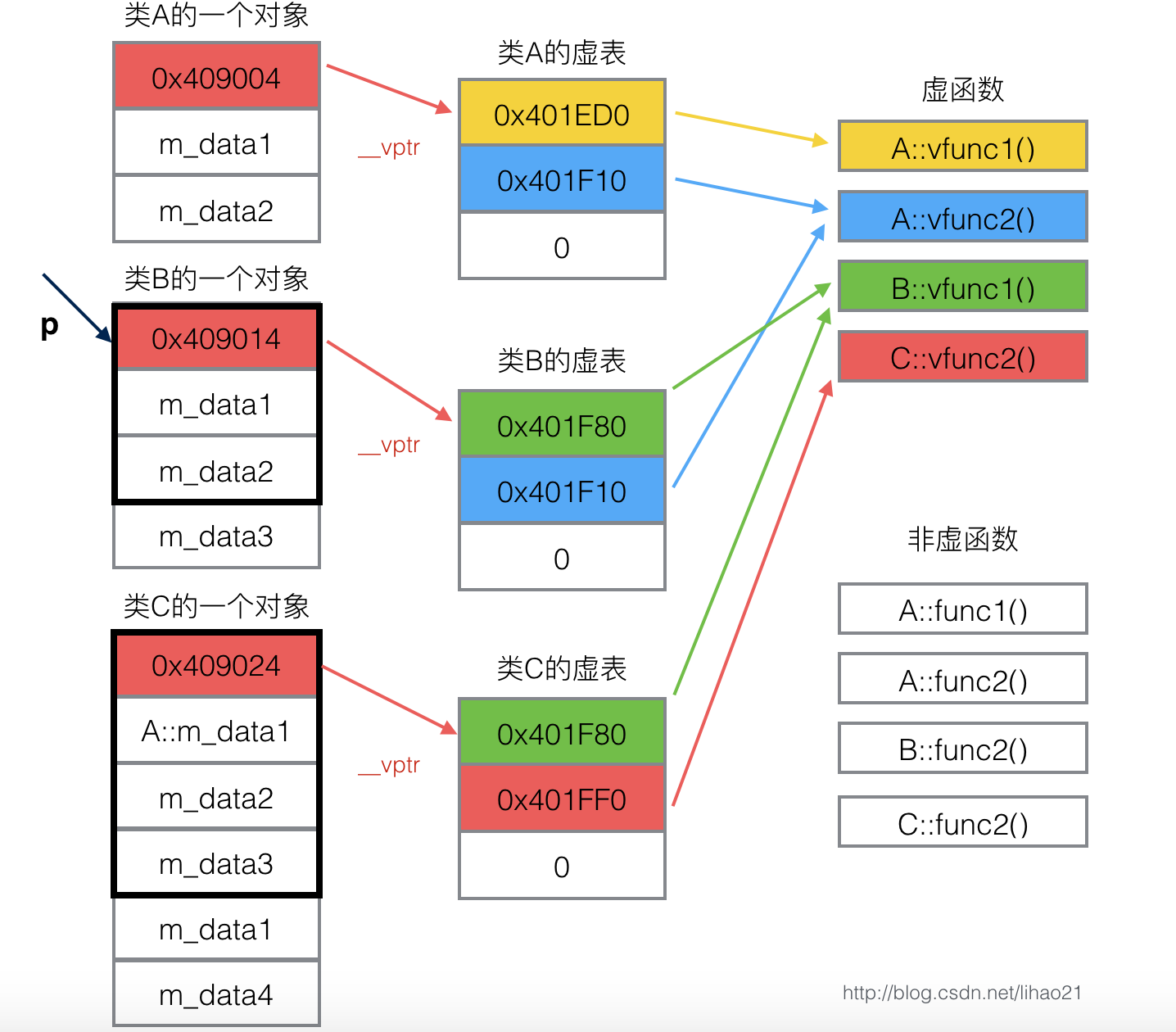``````B bObject;
A* p = &bObject;
p->vfunc1(); //调用B::vfunc1();
``````

• 对象是指针或引用形式
• 静态类型和动态类型不同
• 带虚函数的继承体系